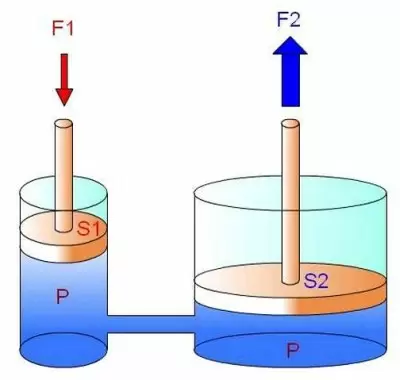# What Is Pascal's Principle? Formula, Law and ExamplesPascal's principle is a law of fluid mechanics. This principle is the basis for the operation of hydraulic systems, and it is formulated as follows:

The pressure exerted on a liquid or gas is transmitted to all fluid points at rest with the same intensity in all directions. In the particles that are in contact with the container, the direction of the pressure exerted by the fluid on the container is perpendicular to its surface.

The French scientist Blaise Pascal formulated the law in 1653 (published in 1663).

Pascal's principle is a consequence of the law of conservation of energy. It is also valid for confined incompressible fluids, compressible fluids (gases), and fluids in a gravity field. However, this principle cannot be applied as a general rule in a moving fluid.

## Pascal's Principle Formula

Pascal's law formula is described below:

P = F / S

Where:

• P is the pressure.

• F is applied force,

• S is the surface to which the force is applied.

Pressure is measured in newtons per square meter, or pascals (Pa), named after the scientist who discovered the law, Pascal.

## Pascal's Law Explained

This formula shows that when a liquid is in contact with two different surfaces, it has repercussions on the forces applied to each of the surfaces.In the illustrated example, we can see a fluid in contact with two different cross-section areas.

At piston number 1, we can formulate that P 1 =F 1 /S 1, and at point 2, we have P 2 =F 2 /S 2.

According to that Pascal's law states, we know that the pressure at a point is equal to the pressure applied to the rest of the liquid. Therefore pressure one and two must be the same P1 = P2.

If we exert a force (F 1 ) on the small piston with a surface (S1), we obtain a pressure that can be compensated on the other larger piston (S2). At the same time, because the surface area (S 2 ) is larger, the fluid exerts a greater resultant force on the piston in order to maintain the F/S ratio.

## Pascal's Experiments

The effects of Pascal's principle were discovered by the French physicist in his famous barrel experiment of 1647.

In the experiment, which also goes by the name "Pascal's paradox," Pascal inserted a 10 m long standpipe into a barrel filled with water. At that moment, Pascal began to pour water into the vertical tube, increasing pressure. The pressure was transmitted equally to the barrel's enclosed fluid and walls until the water broke the barrel due to the intensity accumulated by the water column.

In this experiment, he discovered that pressure depends only on the elevation but not on the size of the fluid container.

### Atmospheric Pressure Experiment

When a barrel is filled with water to a certain level and then tightly plugged, Pascal found that water will not leak out if a small hole is made at the bottom of the barrel.

The reason for this is that the atmospheric pressure is pushing down on the water in the barrel with a force equal to the weight of the water. It is called atmospheric pressure. The water in the barrel is also pushed up on the plug with a force equal to the weight of the water. So two forces are acting on the plug, and they cancel each other out.

## Examples of Pascal's Principle

Pascal's law is the foundation of many mechanical engineering applications that we see in our day to day. Here are some examples of the use and applications of Pascal's principle:

• Hydraulic brake systems allow multiplying the force we exert on the brake to transmit it to the wheels.

• Hydraulic presses use this law to concentrate all the pressure on one point in order to carry out highly concentrated mechanical work on one point.

• A hydraulic jack works using this principle, allowing us to raise a car without effort.

• A hydraulic lift uses the same principle to lift a mass of considerable weight by exerting very little force.

• The arm of an excavator uses Pascal's principle to drive its hydraulic system that multiplies the force from the engine to the shovel.

• Hydraulic cranes use this law to multiply the force generated by their engines and transmit it to the crane arm.

• Hydraulic hammers are used in the construction where a very high force is required to break concrete or stones.

Author:

Published: November 30, 2022
Last review: November 30, 2022